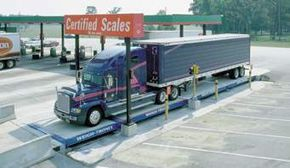# How do truck weigh stations work?­ Most states collect taxes based on the weight ­of transported goods. Truck weigh stations are­ used for these ta­x purposes as well as to monitor the weight of a truck to ensure that it falls within the safety guidelines that each state has in place for its road system.

While the maximum allowed weight varies, a common standard is 34,000 pounds (15,400 kilograms). Weight is usually calculated in two measurements:

• Axle weight - the amount of weight carried by each axle
• Gross weight - the combined weight of all the axles

Truck scales are built to handle an enormous amount of abuse. For example, Weigh-Tronix provides a truck scale that they warranty to weigh trucks with a gross weight of 80,000 lbs (36,000 kg) each at a rate of 200 per day, 365 days a year for 25 years! Truck scales are built out of steel, concrete or, in most cases, a combination of both. The technology used in the scales themselves varies. Let's take a look at some of the more common forms.

Load-cell systems are the most popular technology used. Each cell is comprised of a durable material such as steel or concrete with one or more strain gauges attached to or embedded in it. A strain gauge consists of a wire (or wires) that transmits a mild electric current. As the cell is subjected to weight, the wire in the strain gauge is altered or compressed slightly. The change in the wire results in a difference in the resistance to the current passing through it. The signal from each cell is sent to a junction box, where sensors measure the variance in the current and calculate the amount of weight the scale is supporting.

The strain gauges in load cells can be either compression or tension based. A compression strain gauge is based on how much the cell compresses when pressure is applied, while a tension strain gauge is based on the slight change in shape of the cell caused by the weight.

A bending-plate system uses metal plates with strain gauges attached to them. As weight is applied to the scale, the plates are subjected to stress. The strain gauge on each plate measures the amount of stress and calculates the load required to cause it. The amounts from each gauge are added together to get the total for that axle.

Piezoelectric systems use a series of piezoelectric sensors. The sensors are embedded in a conducting material. When weight is applied, the pressure changes the voltage of the electrical charge flowing through the conductor. The sensors measure the change in voltage and calculate the load. The amounts from each sensor are added together.

In addition to the different scales used, there are at least three methods of getting the weight of a truck:

• One-axle - The most cumbersome method, a truck gradually drives across a single scale, stopping each time a set of wheels is on the scale. Once all the axles have been weighed, the total is added together.
• One-stop - A series of scales are used so that the entire truck can be weighed at once. The scales are typically connected to a single electronic controller that automatically combines the axle weights to get the gross weight.
• Weigh-in-motion (WIM) - A method that is gaining momentum, WIM uses a series of embedded sensors to calculate the weight per axle as a truck drives over the sensor pad. Unlike the other two methods, there is no need for the truck to come to a complete stop while on the scales. In fact, some WIM systems are installed in highways so that all traffic is monitored at speed.